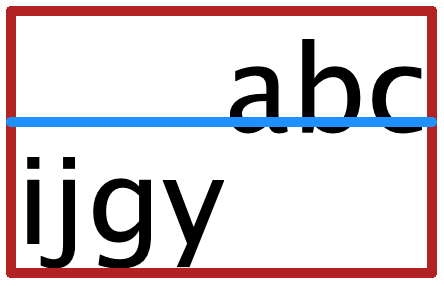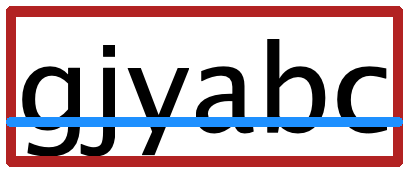6Pict Finders

procedure

(lt-find pict find)
 real? real?
pict : pict-convertible?
find : pict-path?
(ltl-find pict find)
 real? real?
pict : pict-convertible?
find : pict-path?
(lc-find pict find)
 real? real?
pict : pict-convertible?
find : pict-path?
(lbl-find pict find)
 real? real?
pict : pict-convertible?
find : pict-path?
(lb-find pict find)
 real? real?
pict : pict-convertible?
find : pict-path?
(ct-find pict find)
 real? real?
pict : pict-convertible?
find : pict-path?
(ctl-find pict find)
 real? real?
pict : pict-convertible?
find : pict-path?
(cc-find pict find)
 real? real?
pict : pict-convertible?
find : pict-path?
(cbl-find pict find)
 real? real?
pict : pict-convertible?
find : pict-path?
(cb-find pict find)
 real? real?
pict : pict-convertible?
find : pict-path?
(rt-find pict find)
 real? real?
pict : pict-convertible?
find : pict-path?
(rtl-find pict find)
 real? real?
pict : pict-convertible?
find : pict-path?
(rc-find pict find)
 real? real?
pict : pict-convertible?
find : pict-path?
(rbl-find pict find)
 real? real?
pict : pict-convertible?
find : pict-path?
(rb-find pict find)
 real? real?
pict : pict-convertible?
find : pict-path?
Locates a pict designated by find is within pict. If find is a pict, then the pict must have been created as some combination involving find.

If find is a list, then the first element of find must be within pict, the second element of find must be within the second element, and so on.

Examples:
> (define p1 (disk 60))
> (define p2 (rectangle 60 60))
> (define p3 (hc-append p1 p2))
> (define p4 (hc-append p3 (arrow 60 0)))
> (lt-find p4 p1)
 0 0
> (cb-find p4 p2)
 90 60
> (rb-find p3 p1)
 60 60
> (lt-find p4 (list p1))
 0 0
> (lt-find p4 (list p2 p1))

> (lt-find p4 (list p2))
 60 0
> (lt-find p4 (list p3 p2))
 60 0
 > (pin-over p4 p2 lt-find (colorize (text "lt-find") "darkgreen"))> (panorama (pin-over p4 p2 rb-find (colorize (text "rb-find") "darkgreen")))Changed in version 1.11 of package pict-lib: Removed implicit truncation of some centered coordinates to integers.

 procedure v : any/c
Returns #t if v is a pict-convertible? or a non-empty list of pict-convertible?s.

Examples:
 > (pict-path? null) #f > (pict-path? (disk 30)) #t > (pict-path? (list (disk 30) (rectangle 10 10))) #t

 procedure(launder pict) → pict? pict : pict-convertible?
Creates a pict that has the same drawing and bounding box of pict, but which hides all of its sub-picts so that they cannot be found with functions like lt-find. If pict has a last-line pict, then the laundered pict has a fresh last-line pict with the same shape and location.

Examples:
 > (define p1 (disk 60)) > (define p2 (rectangle 60 60)) > (define p3 (hc-append p1 p2)) > (lt-find (launder p4) p3) find-XX: sub-pict: # not found in: #